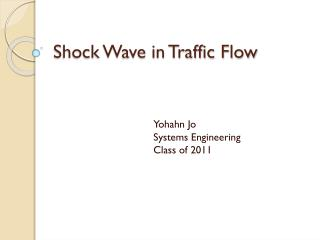DownloadDownload PresentationShock Wave in Traffic Flow

# Shock Wave in Traffic Flow

Télécharger la présentation## Shock Wave in Traffic Flow

- - - - - - - - - - - - - - - - - - - - - - - - - - - E N D - - - - - - - - - - - - - - - - - - - - - - - - - - -
##### Presentation Transcript

1. Shock Wave in Traffic Flow Yohahn Jo Systems Engineering Class of 2011

2. Background • Traffic waves work very similar to shock waves • In a traffic jam, traffic wave moves backward http://www.youtube.com/watch?v=Suugn-p5C1M

3. How Do We Determine Shock Wave Speeds? • Usw = speed of shock wave (km/h or mph) • qi = density (vehicles per hour) • ki = flow (vehicles per kilometer(or miles)) • Positive usw = shock wave moving in the same direction as flow • Negative usw = shock wave moving opposite

4. Example • traffic instantaneously changes from state B to state A where state B is up stream and has a volume of 1200 vph and 40 mph and state A is 2000 vph at 20 mph. The resulting shock wave (the difference between the speed of the forward moving traffic and the backward moving platoon of traffic is defined by the equation • Apply the equation: • Qa = 2000 vph, ka = 2000 vph/20 mph = 100 vph • Qb = 1200 vph, kb = 1200 vph/40 mph = 30 vph • Usw = (2000 – 1200)/(100 - 30) = 11.4 mph

5. Greenshield’s Model • Bruce D. Greenshields (1935) • Linear relationship between speed and density u = velocity uf = velocity of free flow kj = jam density k = density

6. Example • a traffic stream on the approach to a signal has an approach speed of 40 mph and density of 45 vpm and a jam density of 130 vpm. Find a speed of the shock wave. • k = 45 vpm • kj = 130 vpm • u = 40 mph

7. 40 = uf(1 – 45/130) • uf = 61.2 mph • u = q/ k • q = 45 * 40 = 1800 vph • usw = (0 – 1800)/(130 – 45) = -21.2 mph

8. Problems with Greenshield’s Model • Does not consider human factors or look at individual cars • Based on single lane roads

9. Questions

10. Sources • www.cee.mtu.edu/~balkire/ce3401tc/ce3401Lec39.doc • http://www.newscientist.com/article/dn13402 • http://www.webs1.uidaho.edu/niatt_labmanual/Chapters/trafficflowtheory/theoryandconcepts/ShockWaves.htm • http://mikeywally.wordpress.com/category/traffic-flow/ • http://nptel.iitm.ac.in/courses/Webcourse-contents/IIT-KANPUR/transport_e/TransportationII/lecture8/text/4slide.htm • http://books.google.com/books?id=Zut3O7jVIiUC&pg=PA11&lpg=PA11&dq=greenshield%27s+model+traffic&source=bl&ots=fbwtDUcxVt&sig=Ar8M0sTSQIhZ_VvredrXa2nlqMs&hl=en&ei=6pD5SsKsJY7CNt656M0K&sa=X&oi=book_result&ct=result&resnum=3&ved=0CBAQ6AEwAg#v=onepage&q=&f=false